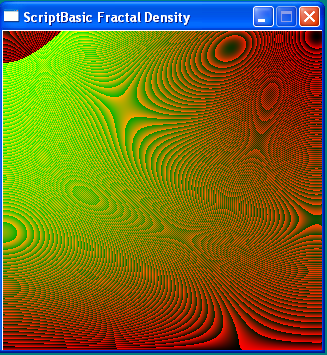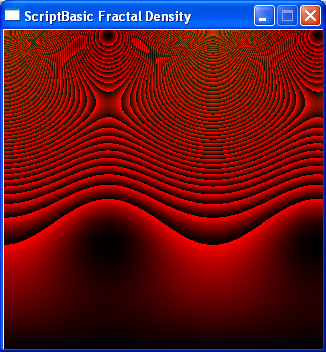###Author Topic: Geoff Anderson Fractal Density Theory  (Read 2815 times)

####John##### Geoff Anderson Fractal Density Theory
« on: March 09, 2013, 08:13:59 PM »
Quote
This is an intriguing theory of everything, that requires little more than Newtonian dynamics and an understanding of fractals. It scales in concept from subatomic particles to galactic clusters.

CharlesI attached the example Charles posted if you would like to see this in something other than  SB.

Code: [Select]
`' Geoff Anderson Fractal Density TheoryDECLARE SUB DLLC_File ALIAS "dllfile" LIB "DLLC"DECLARE SUB DLLC_Proc ALIAS "dllproc" LIB "DLLC"DECLARE SUB DLLC_Call ALIAS "dllcall" LIB "DLLC"sw = DLLC_File("sw.dll")Window = DLLC_Proc(sw, "Window i = (i width, i height, i mode)")SetCaption = DLLC_Proc(sw, "SetCaption i = (c*capText)")SetPixel = DLLC_Proc(sw, "SetPixel i = (i xPos, i yPos, i color)")Key = DLLC_Proc(sw, "Key i = (i mKey)")Redraw = DLLC_Proc(sw, "Redraw ( )")CloseWindow = DLLC_Proc(sw, "CloseWindow i = ( )")DLLC_Call(Window, 320, 320, 1)DLLC_Call(SetCaption,"ScriptBasic Fractal Density")SUB Trauma(im, ii, st) LOCAL fx, fy, i LOCAL r, g, b, c, x, y FOR x = 0 TO 319   i = 1    fy = 0   FOR y = 0 TO 319     c = i * (1 + COS(fx)) + i * (1 + COS(fy))     IF c <> undef THEN       DLLC_Call(SetPixel, x, 320 - y, c)     END IF     i = i * im + ii     fy += st   NEXT   fx += st  NEXTEND SUBWHILE DLLC_Call(Key, 27) = 0  m = 1  n =1  FOR i = 1 TO 120    Trauma(m, n, .01)    n += 111    DLLC_Call(Redraw)  NEXT  m = 1.0100  n = .4  FOR i = 1 TO 120    Trauma(m, n, .03)    m += .0005     DLLC_Call(Redraw)  NEXTWENDDLLC_Call(CloseWindow)`
« Last Edit: March 09, 2013, 10:56:15 PM by JRS »